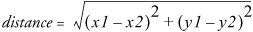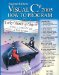# Wrap-Up

### Exercises

 15.3 (Bouncing Ball) Write a program that bounces a blue ball inside a Panel. The ball should begin moving with a MouseClick event. When the ball hits the edge of the Panel, it should bounce off the edge and continue in the opposite direction. The ball's position should be updated using a THRead and redrawn periodically using another THRead. Use float variables to maintain the ball's position, radius and velocity. Draw the ball using the FillEllipse method of class Graphics (see Fig. 13.38). Remember to terminate all Threads when the user closes the application. 15.4 (Enhanced Bouncing Ball) Modify the program in Exercise 15.3 to add a new ball each time the user clicks the mouse. Provide for a maximum of 10 balls. Use a separate THRead to control the movements of each ball, plus an additional Thread to periodically redraw all of the balls. Randomly choose the color and size for each new ball. 15.5 (Bouncing Balls with Shadows) Modify the program in Exercise 15.4 to add shadows. As a ball moves, draw a solid black oval at the bottom of the Panel. You may consider adding a 3-D effect by increasing or decreasing the size of the shadow depending upon the vertical position of the ball. 15.6 (Bouncing Balls with Collision Detection) Modify the program in Exercise 15.4 or Exercise 15.5 to bounce the balls off each other when they collide. A collision should occur between two balls when the distance between the centers of those two balls is less than the sum of the two balls' radii. When a collision between two balls occurs, use the following equations to modify each ball's velocity:overlap = ( radius1 + radius2 ) distance xVelocity1 = xVelocity1 + ( x1 x2 ) * ( overlap / distance ) * 0.5 yVelocity1 = yVelocity1 + ( y1 y2 ) * ( overlap / distance ) * 0.5 xVelocity2 = xVelocity2 + ( x2 x1 ) * ( overlap / distance ) * 0.5 yVelocity2 = yVelocity2 + ( y2 y1 ) * ( overlap / distance ) * 0.5 [Note: Ensure that distance does not equal zero.]

## Strings, Characters and Regular ExpressionsVisual C# 2005 How to Program (2nd Edition)
ISBN: 0131525239
EAN: 2147483647
Year: 2004
Pages: 600